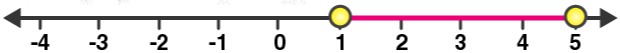# Selina Solutions Concise Maths Class 10 Chapter 4 Linear Inequations (In one variable) Exercise 4(B)

After solving a linear inequation, the representation of the solution on the number line is discussed in this exercise. The concept of solving combined inequations is also discussed in the same exercise. The Selina Solutions for Class 10 Maths has solutions to these questions which are prepared by experts at BYJU’S. The solutions of this particular exercise problems are available in the Concise Selina Solutions for Class 10 Maths Chapter 4 Linear Inequations (In one variable) Exercise 4(B) PDF provided below.

## Selina Solutions Concise Maths Class 10 Chapter 4 Linear Inequations (In one variable) Exercise 4(B) Download PDF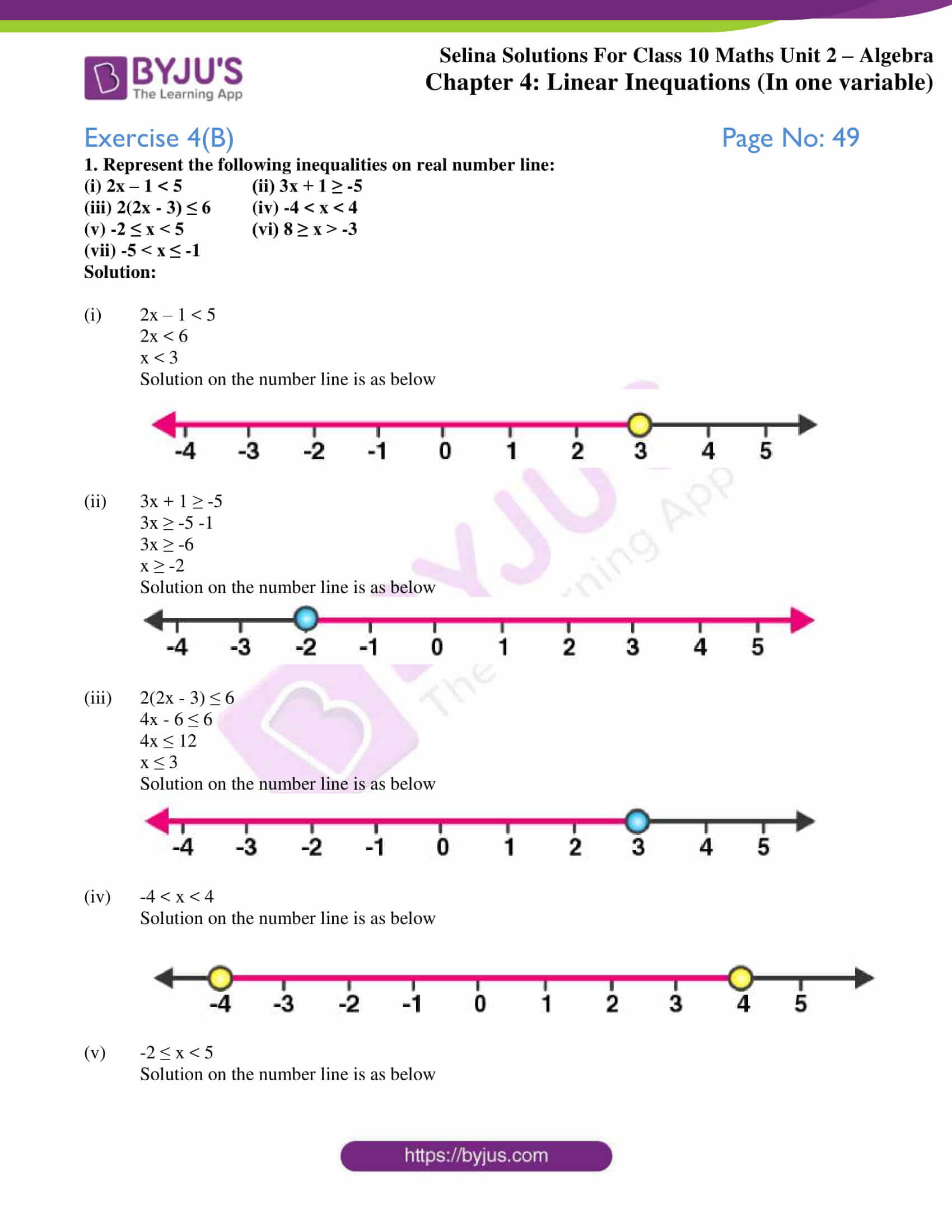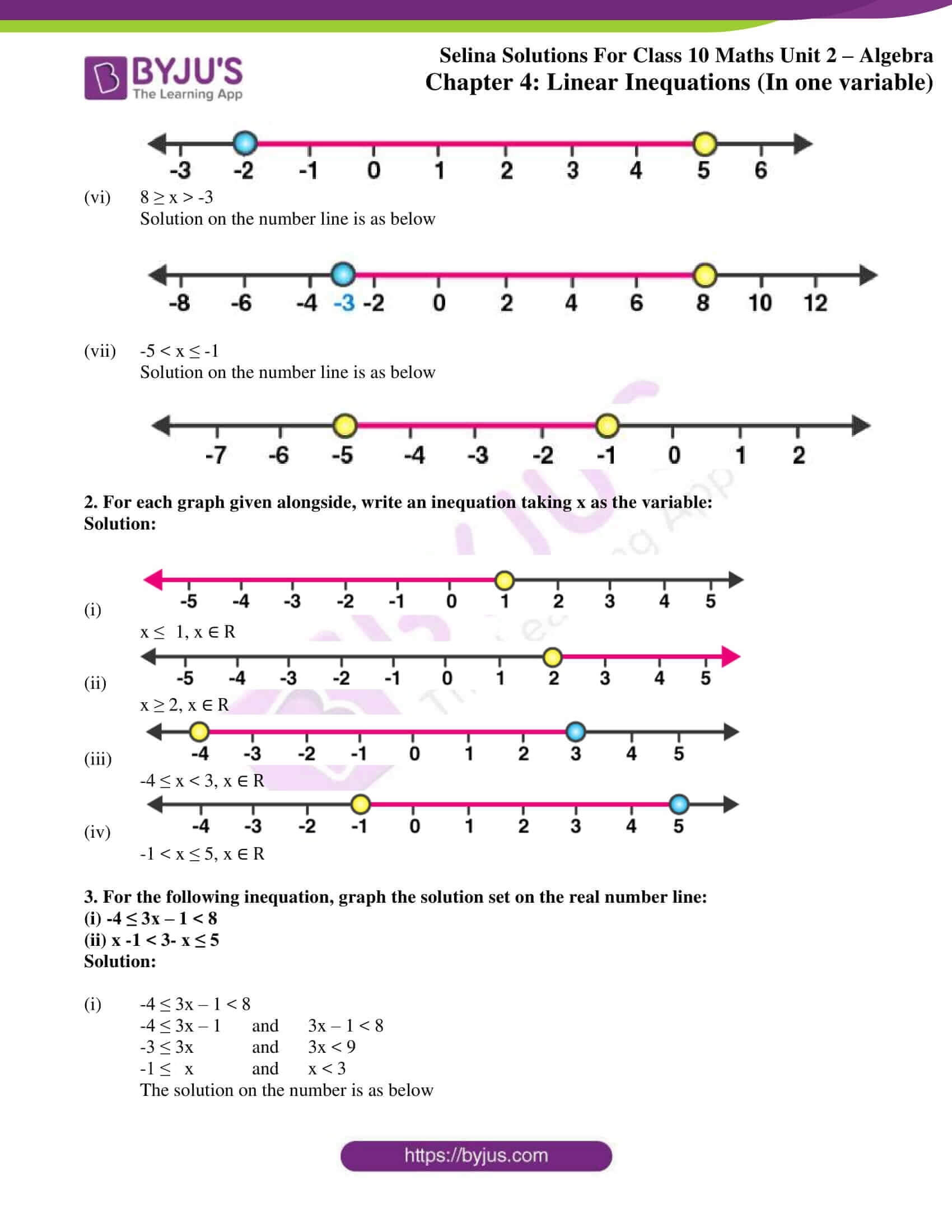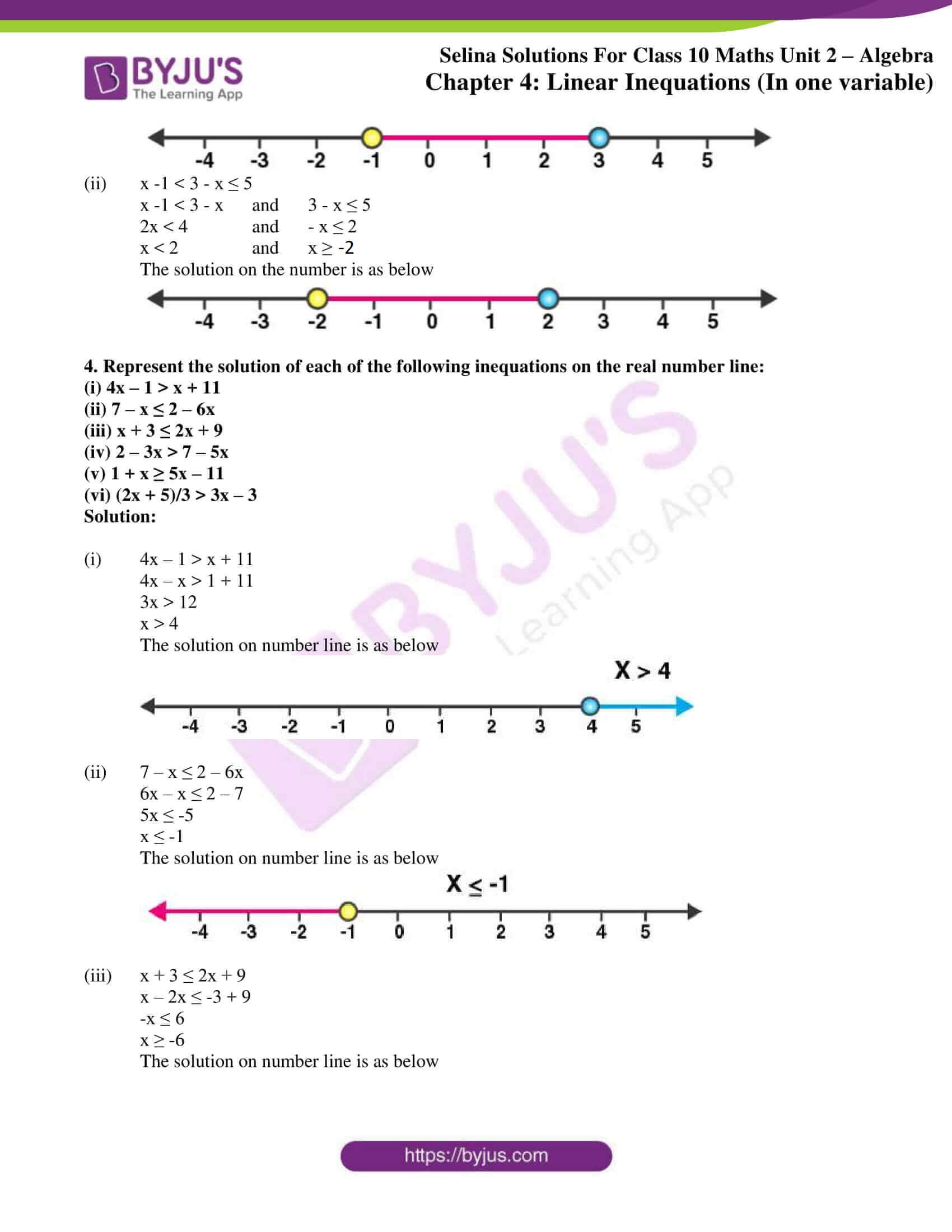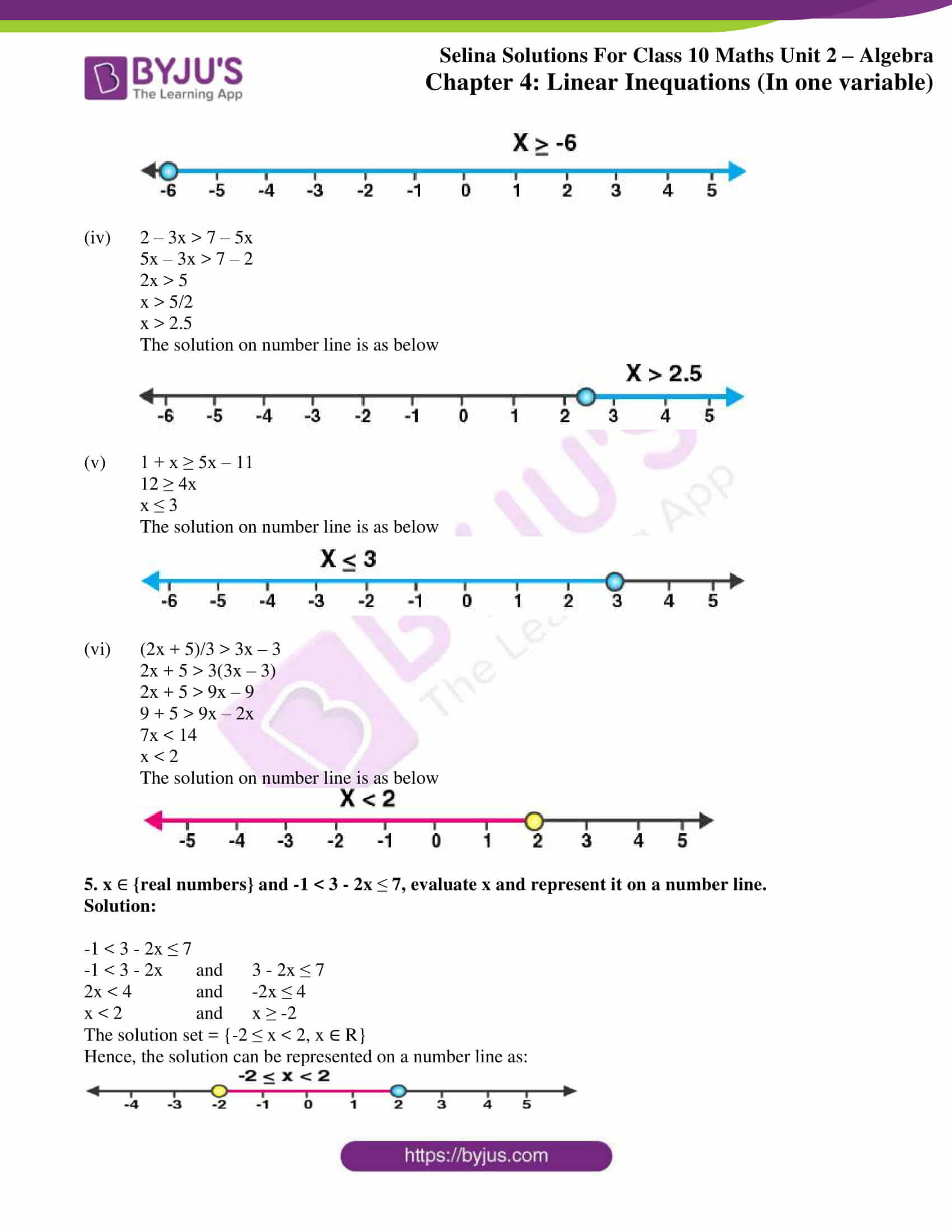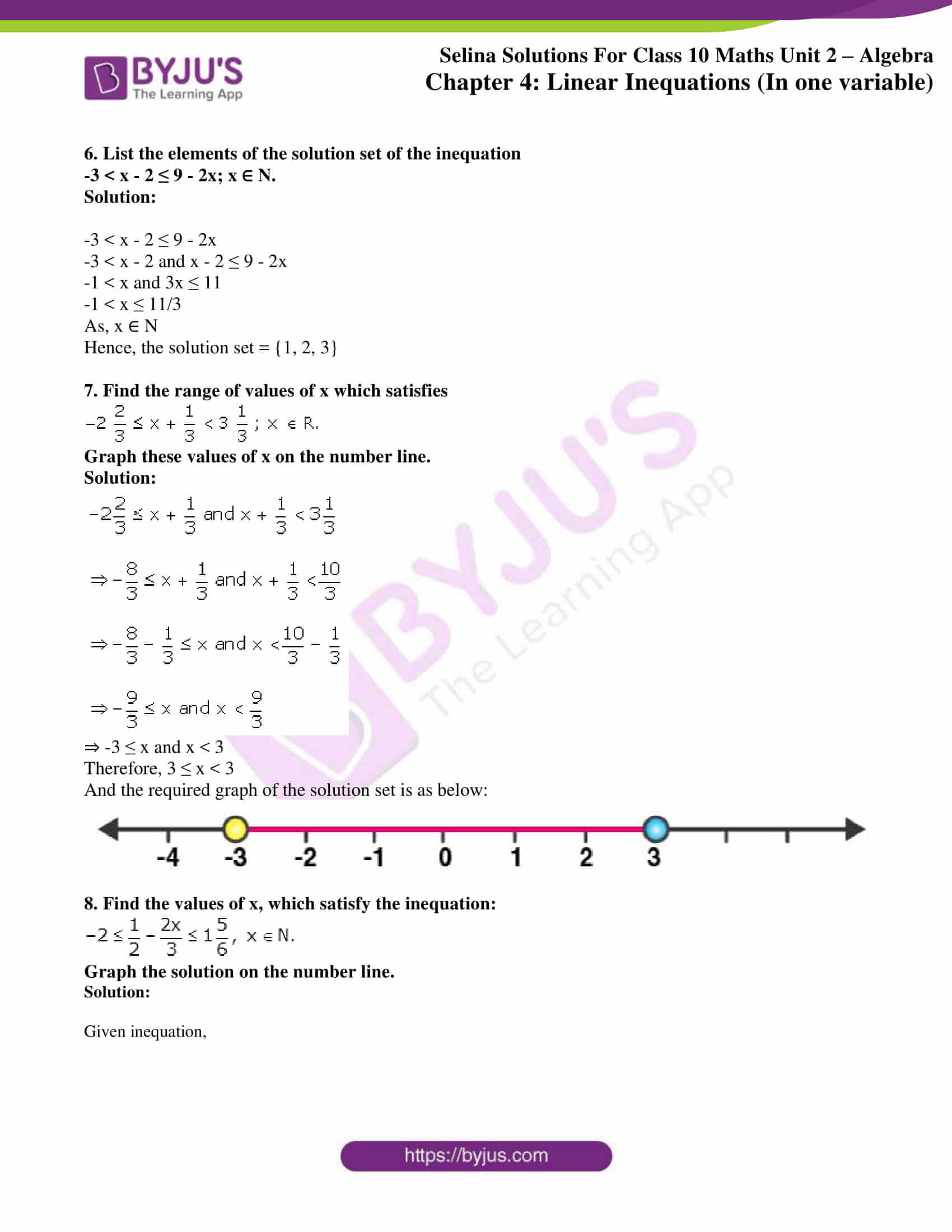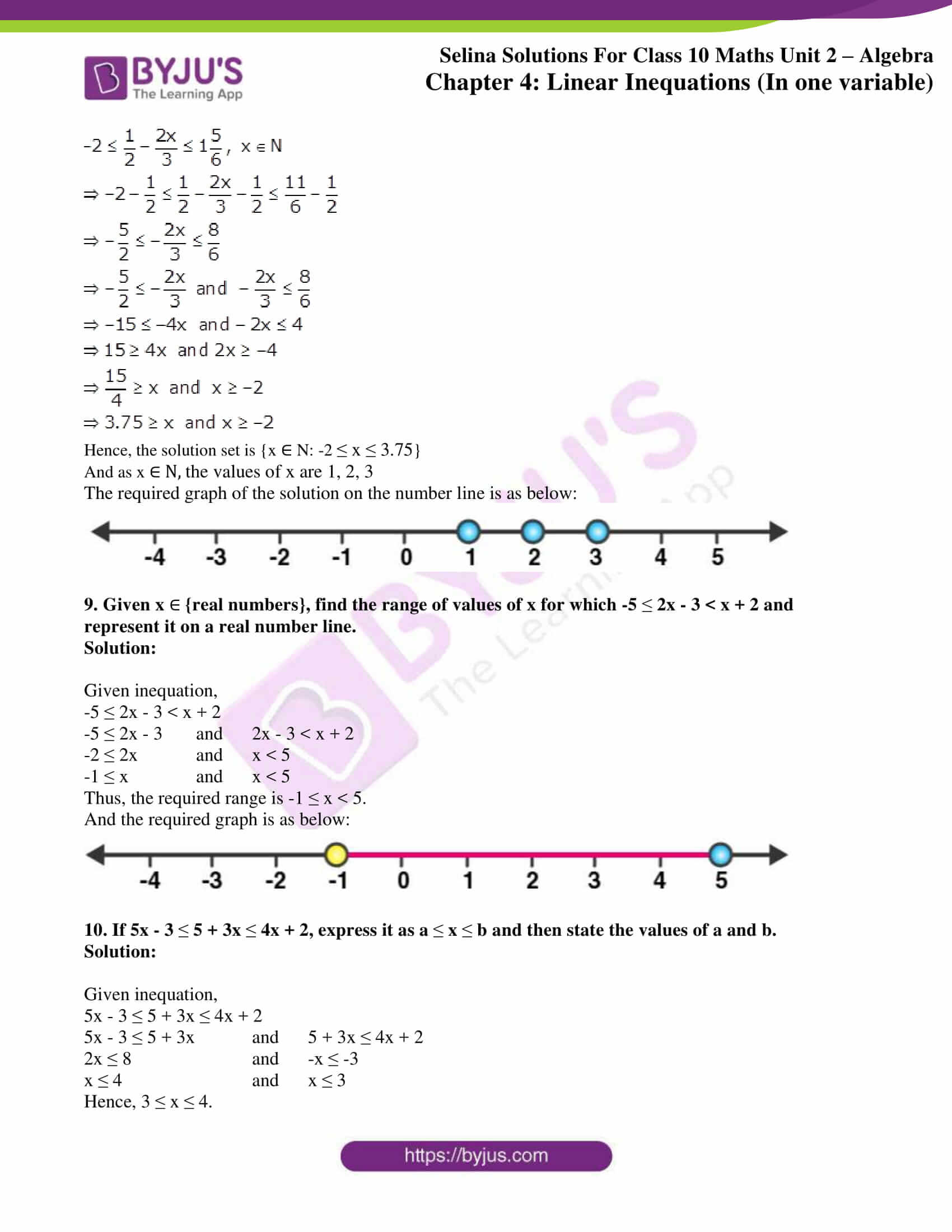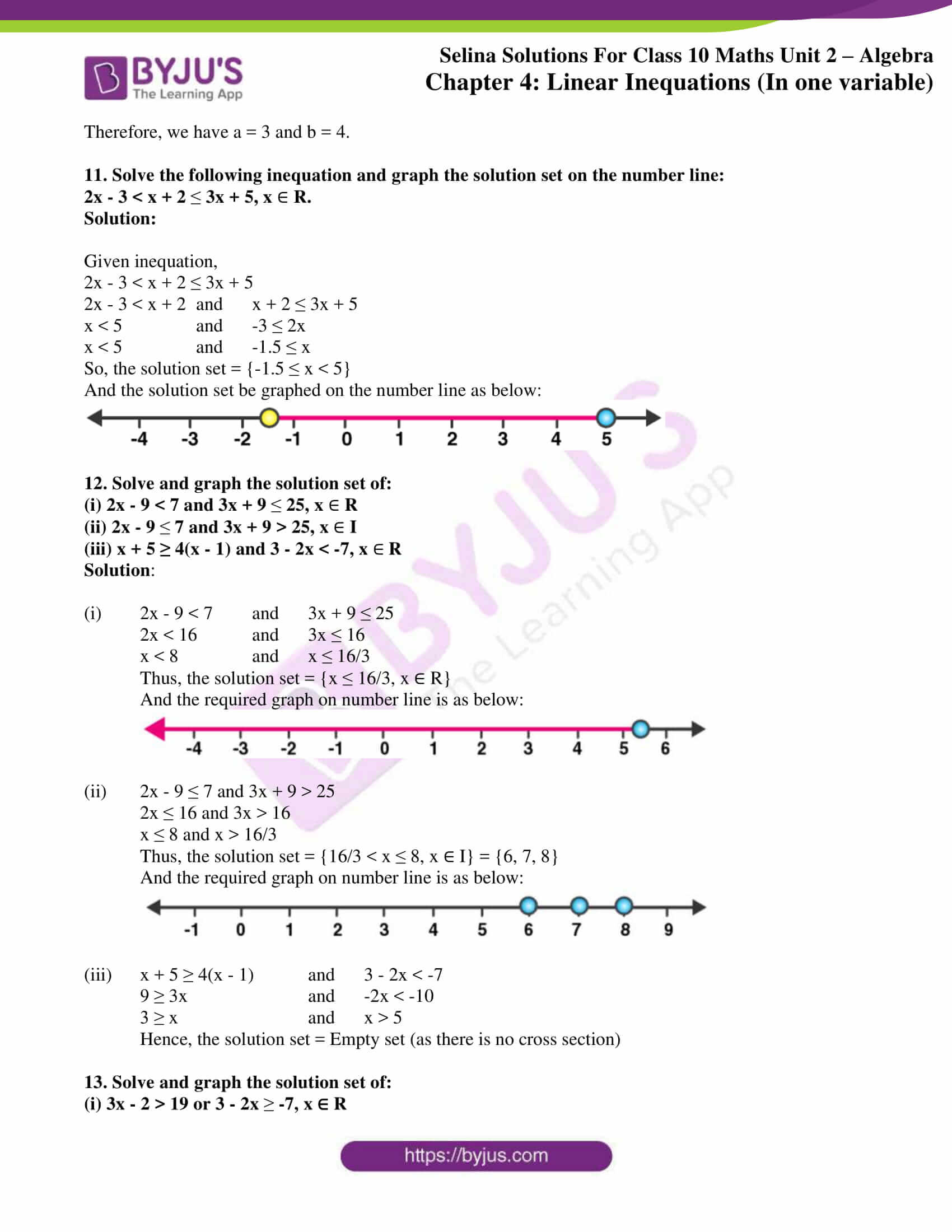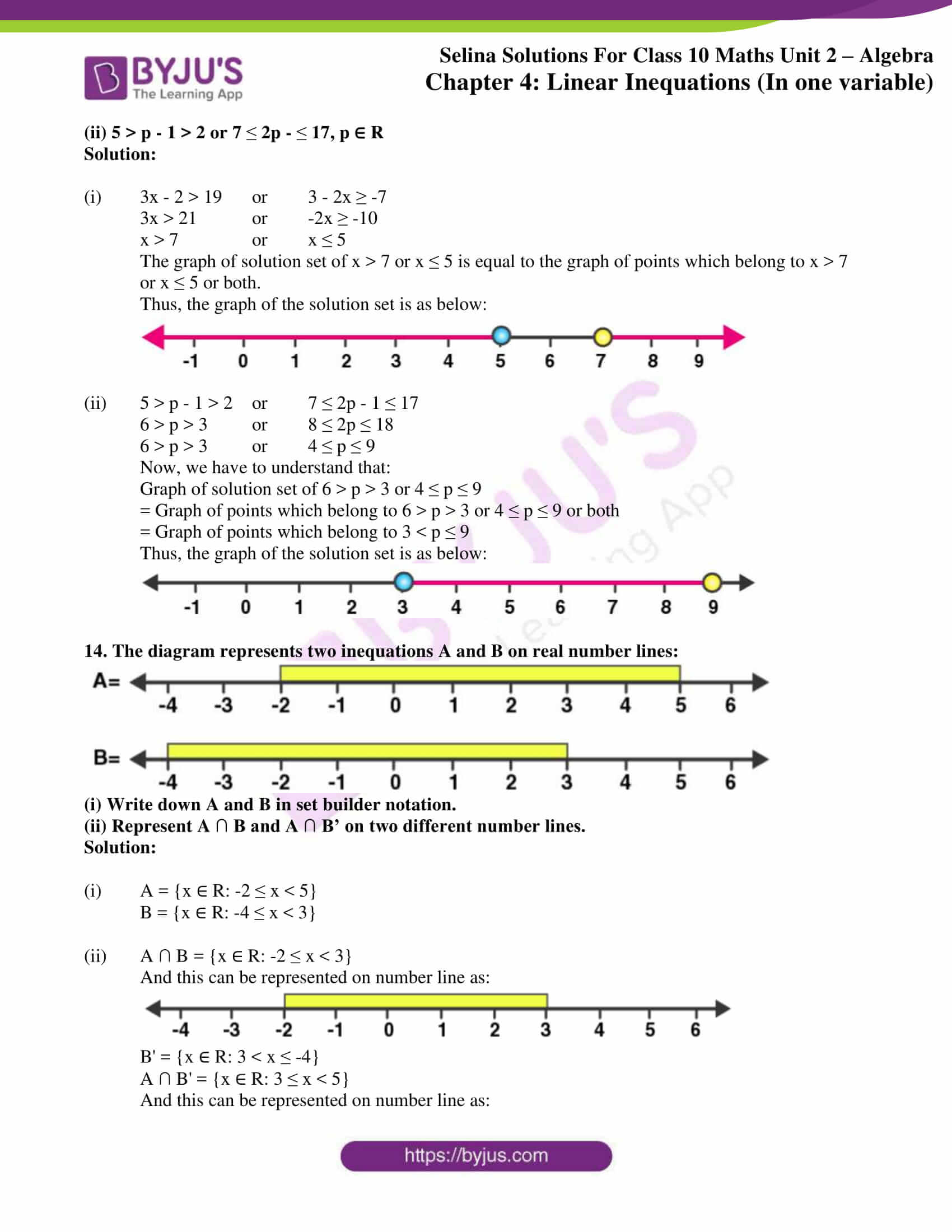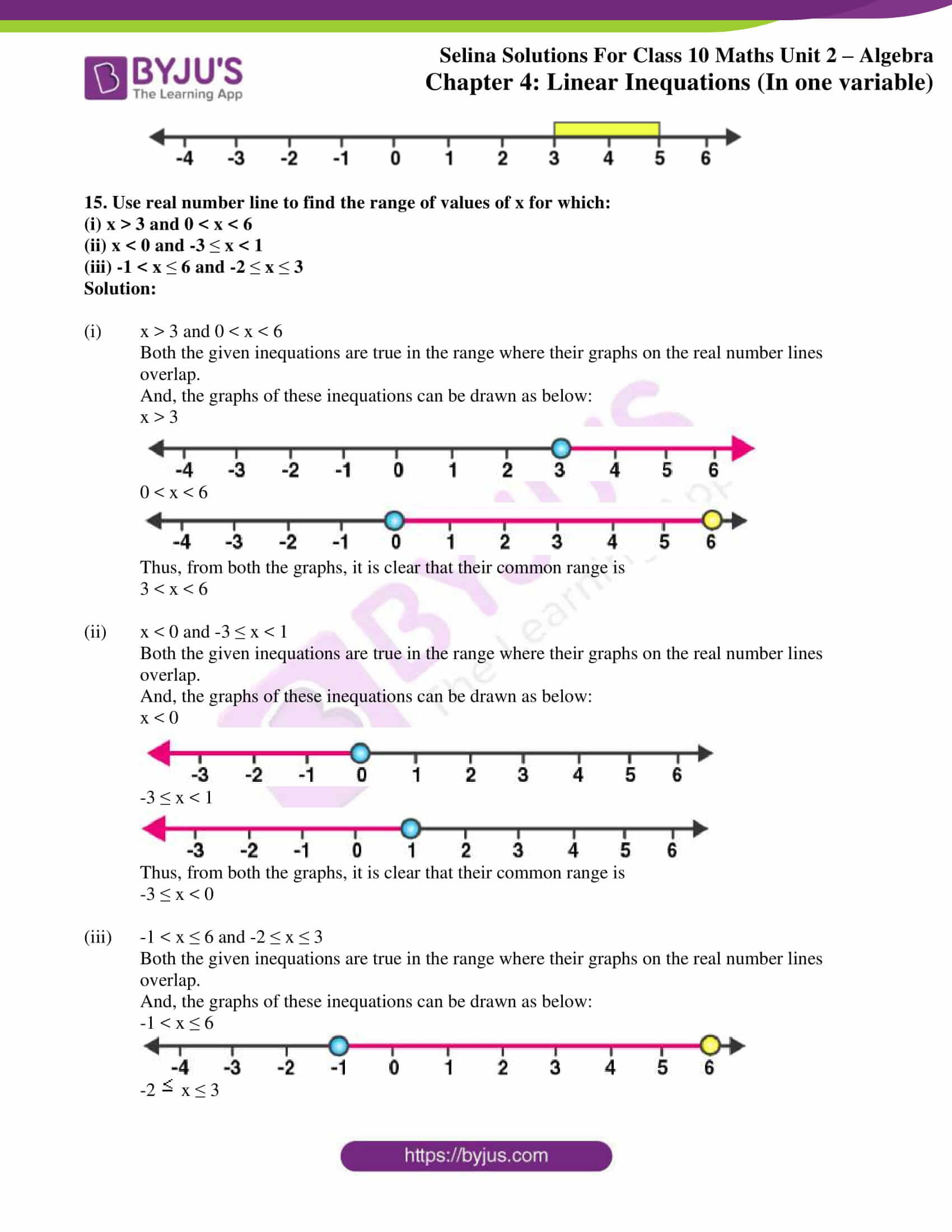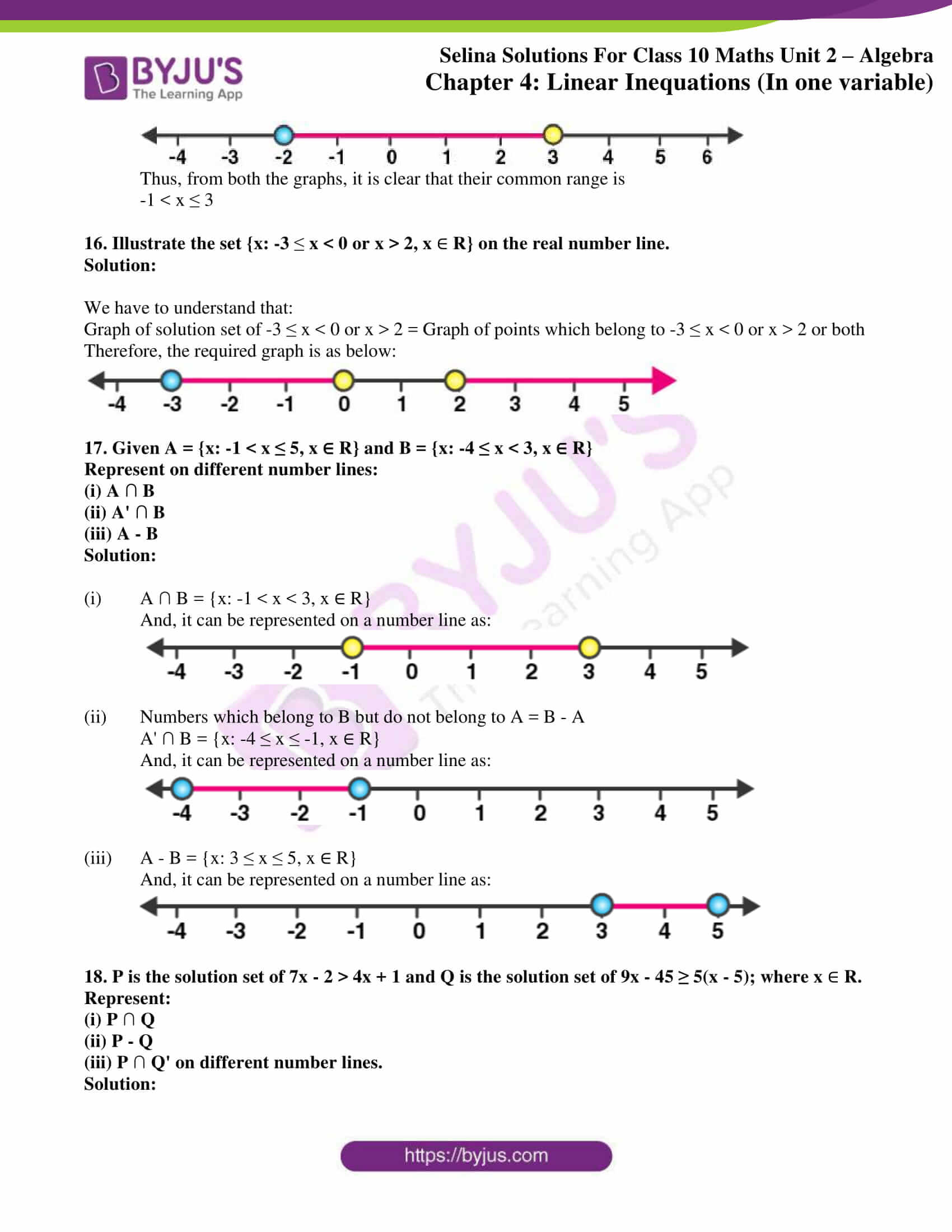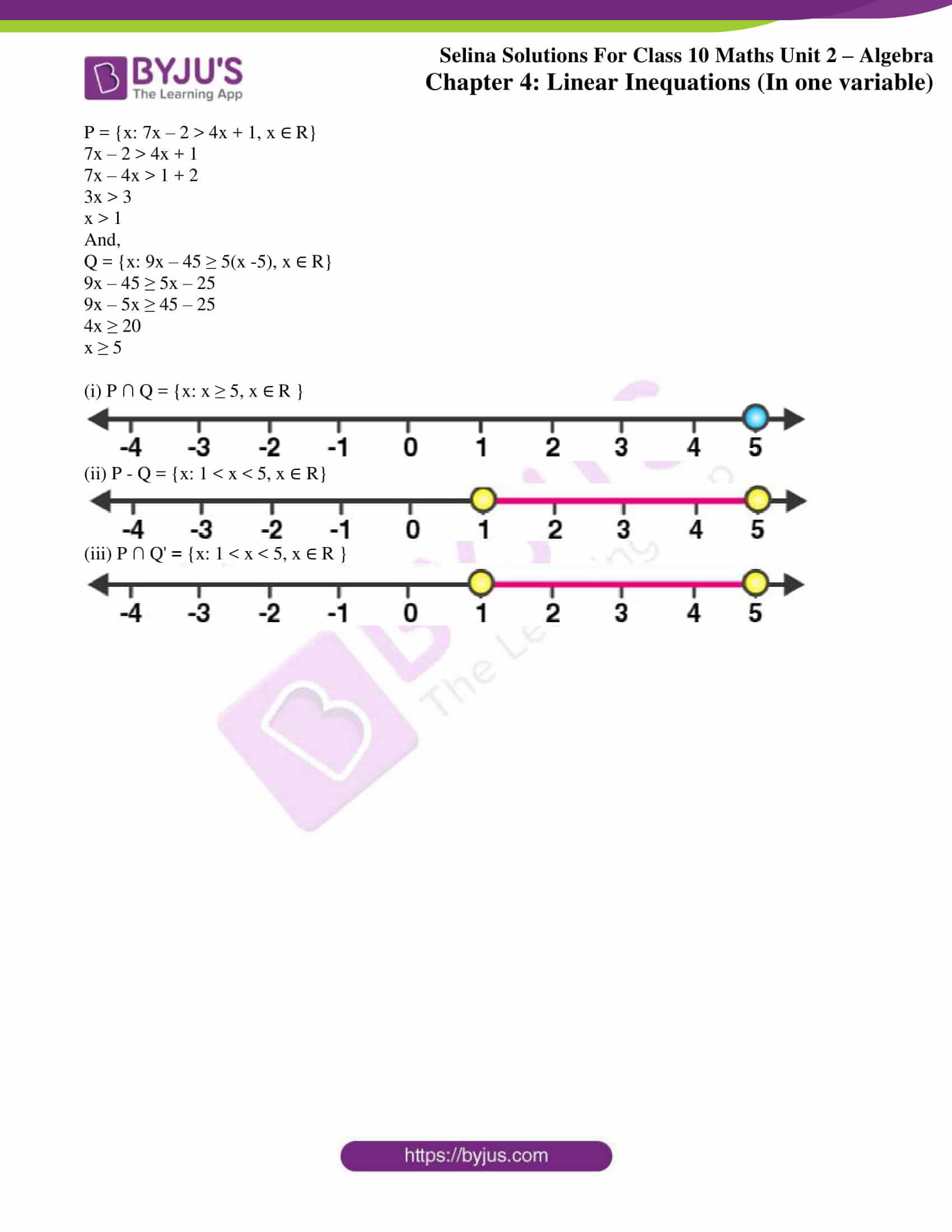### Access another exercise of Selina Solutions Concise Maths Class 10 Chapter 4 Linear Inequations (In one variable)

Exercise 4(A) Solutions

### Access Concise Selina Solutions for Class 10 Maths Chapter 4 Linear Inequations (In one variable) Exercise 4(B)

1. Represent the following inequalities on real number line:

(i) 2x – 1 < 5 (ii) 3x + 1 ≥ -5

(iii) 2(2x – 3) ≤ 6 (iv) -4 < x < 4

(v) -2 ≤ x < 5 (vi) 8 ≥ x > -3

(vii) -5 < x ≤ -1

Solution:

(i) 2x – 1 < 5

2x < 6

x < 3

Solution on the number line is as below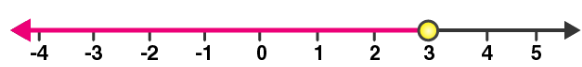(ii) 3x + 1 ≥ -5

3x ≥ -5 -1

3x ≥ -6

x ≥ -2

Solution on the number line is as below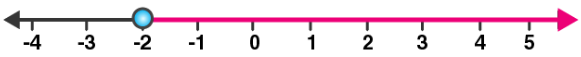(iii) 2(2x – 3) ≤ 6

4x – 6 ≤ 6

4x ≤ 12

x ≤ 3

Solution on the number line is as below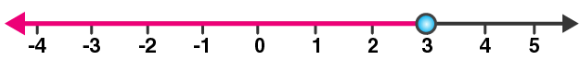(iv) -4 < x < 4

Solution on the number line is as below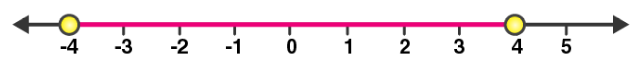(v) -2 ≤ x < 5

Solution on the number line is as below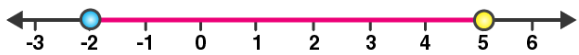(vi) 8 ≥ x > -3

Solution on the number line is as below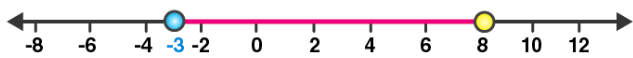(vii) -5 < x ≤ -1

Solution on the number line is as below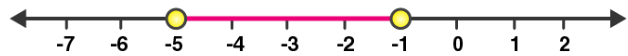2. For each graph given alongside, write an inequation taking x as the variable:

Solution:

(i)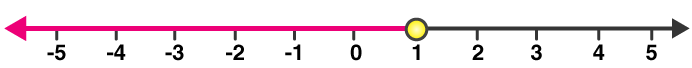x ≤ 1, x ∈ R

(ii)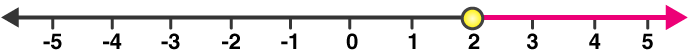x ≥ 2, x ∈ R

(iii)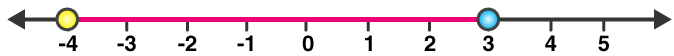-4 ≤ x < 3, x ∈ R

(iv)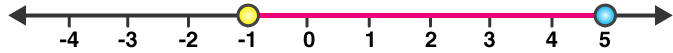-1 < x ≤ 5, x ∈ R

3. For the following inequation, graph the solution set on the real number line:

(i) -4 ≤ 3x – 1 < 8

(ii) x -1 < 3- x ≤ 5

Solution:

(i) -4 ≤ 3x – 1 < 8

-4 ≤ 3x – 1 and 3x – 1 < 8

-3 ≤ 3x and 3x < 9

-1 ≤ x and x < 3

The solution on the number is as below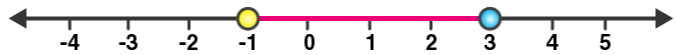(ii) x -1 < 3 – x ≤ 5

x -1 < 3 – x and 3 – x ≤ 5

2x < 4 and – x ≤ 2

x < 2 and x ≥ -2

The solution on the number is as below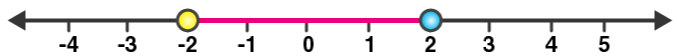4. Represent the solution of each of the following inequations on the real number line:

(i) 4x – 1 > x + 11

(ii) 7 – x ≤ 2 – 6x

(iii) x + 3 ≤ 2x + 9

(iv) 2 – 3x > 7 – 5x

(v) 1 + x ≥ 5x – 11

(vi) (2x + 5)/3 > 3x – 3

Solution:

(i) 4x – 1 > x + 11

4x – x > 1 + 11

3x > 12

x > 4

The solution on number line is as below(ii) 7 – x ≤ 2 – 6x

6x – x ≤ 2 – 7

5x ≤ -5

x ≤ -1

The solution on number line is as below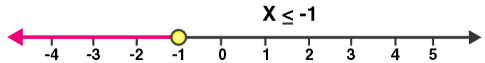(iii) x + 3 ≤ 2x + 9

x – 2x ≤ -3 + 9

-x ≤ 6

x ≥ -6

The solution on number line is as below(iv) 2 – 3x > 7 – 5x

5x – 3x > 7 – 2

2x > 5

x > 5/2

x > 2.5

The solution on number line is as below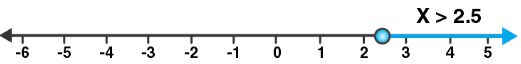(v) 1 + x ≥ 5x – 11

12 ≥ 4x

x ≤ 3

The solution on number line is as below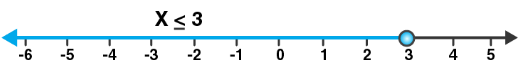(vi) (2x + 5)/3 > 3x – 3

2x + 5 > 3(3x – 3)

2x + 5 > 9x – 9

9 + 5 > 9x – 2x

7x < 14

x < 2

The solution on number line is as below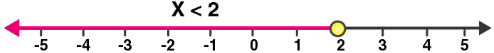5. x  {real numbers} and -1 < 3 – 2x  7, evaluate x and represent it on a number line.

Solution:

-1 < 3 – 2x ≤ 7

-1 < 3 – 2x and 3 – 2x ≤ 7

2x < 4 and -2x ≤ 4

x < 2 and x ≥ -2

The solution set = {-2 ≤ x < 2, x ∈ R}

Hence, the solution can be represented on a number line as: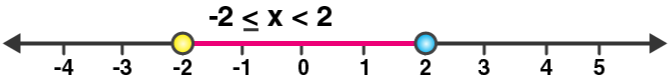6. List the elements of the solution set of the inequation

-3 < x – 2 ≤ 9 – 2x; x ∈ N.

Solution:

-3 < x – 2 ≤ 9 – 2x

-3 < x – 2 and x – 2 ≤ 9 – 2x

-1 < x and 3x ≤ 11

-1 < x ≤ 11/3

As, x ∈ N

Hence, the solution set = {1, 2, 3}

7. Find the range of values of x which satisfies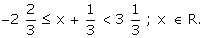Graph these values of x on the number line.

Solution: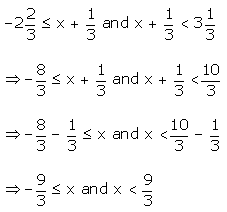⇒ -3 ≤ x and x < 3

Therefore, 3 ≤ x < 3

And the required graph of the solution set is as below: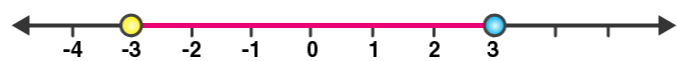8. Find the values of x, which satisfy the inequation: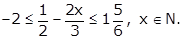Graph the solution on the number line.

Solution:

Given inequation,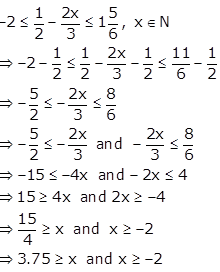Hence, the solution set is {x ∈ N: -2 ≤ x ≤ 3.75}

And as x ∈ N, the values of x are 1, 2, 3

The required graph of the solution on the number line is as below: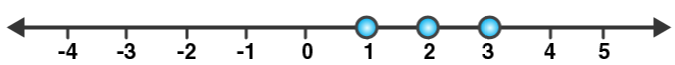9. Given x {real numbers}, find the range of values of x for which -5  2x – 3 < x + 2 and represent it on a real number line.

Solution:

Given inequation,

-5 ≤ 2x – 3 < x + 2

-5 ≤ 2x – 3 and 2x – 3 < x + 2

-2 ≤ 2x and x < 5

-1 ≤ x and x < 5

Thus, the required range is -1 ≤ x < 5.

And the required graph is as below: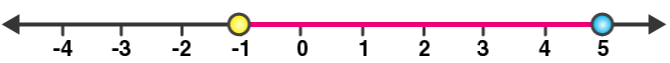10. If 5x – 3  5 + 3x  4x + 2, express it as a  x  b and then state the values of a and b.

Solution:

Given inequation,

5x – 3 ≤ 5 + 3x ≤ 4x + 2

5x – 3 ≤ 5 + 3x and 5 + 3x ≤ 4x + 2

2x ≤ 8 and -x ≤ -3

x ≤ 4 and x ≤ 3

Hence, 3 ≤ x ≤ 4.

Therefore, we have a = 3 and b = 4.

11. Solve the following inequation and graph the solution set on the number line:

2x – 3 < x + 2  3x + 5, x  R.

Solution:

Given inequation,

2x – 3 < x + 2 ≤ 3x + 5

2x – 3 < x + 2 and x + 2 ≤ 3x + 5

x < 5 and -3 ≤ 2x

x < 5 and -1.5 ≤ x

So, the solution set = {-1.5 ≤ x < 5}

And the solution set be graphed on the number line as below: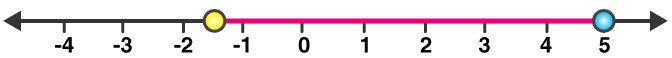12. Solve and graph the solution set of:

(i) 2x – 9 < 7 and 3x + 9  25, x  R

(ii) 2x – 9  7 and 3x + 9 > 25, x  I

(iii) x + 5 ≥ 4(x – 1) and 3 – 2x < -7, x R

Solution:

(i) 2x – 9 < 7 and 3x + 9 ≤ 25

2x < 16 and 3x ≤ 16

x < 8 and x ≤ 16/3

Thus, the solution set = {x ≤ 16/3, x ∈ R}

And the required graph on number line is as below: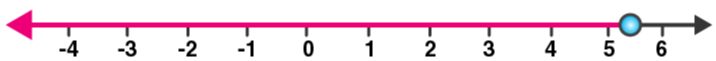(ii) 2x – 9 ≤ 7 and 3x + 9 > 25

2x ≤ 16 and 3x > 16

x ≤ 8 and x > 16/3

Thus, the solution set = {16/3 < x ≤ 8, x ∈ I} = {6, 7, 8}

And the required graph on number line is as below: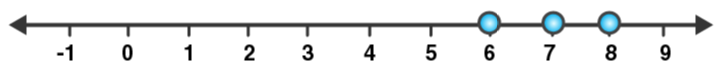(iii) x + 5 ≥ 4(x – 1) and 3 – 2x < -7

9 ≥ 3x and -2x < -10

3 ≥ x and x > 5

Hence, the solution set = Empty set (as there is no cross section)

13. Solve and graph the solution set of:

(i) 3x – 2 > 19 or 3 – 2x  -7, x ∈ R

(ii) 5 > p – 1 > 2 or 7  2p –  17, p ∈ R

Solution:

(i) 3x – 2 > 19 or 3 – 2x ≥ -7

3x > 21 or -2x ≥ -10

x > 7 or x ≤ 5

The graph of solution set of x > 7 or x ≤ 5 is equal to the graph of points which belong to x > 7 or x ≤ 5 or both.

Thus, the graph of the solution set is as below: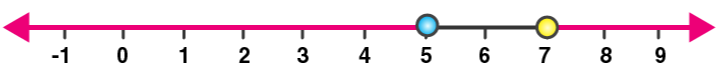(ii) 5 > p – 1 > 2 or 7 ≤ 2p – 1 ≤ 17

6 > p > 3 or 8 ≤ 2p ≤ 18

6 > p > 3 or 4 ≤ p ≤ 9

Now, we have to understand that:

Graph of solution set of 6 > p > 3 or 4 ≤ p ≤ 9

= Graph of points which belong to 6 > p > 3 or 4 ≤ p ≤ 9 or both

= Graph of points which belong to 3 < p ≤ 9

Thus, the graph of the solution set is as below:14. The diagram represents two inequations A and B on real number lines: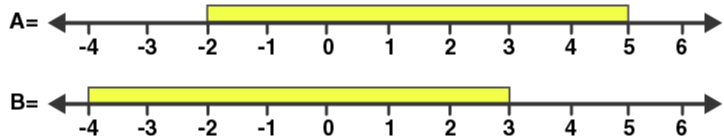(i) Write down A and B in set builder notation.

(ii) Represent A ∩ B and A ∩ B’ on two different number lines.

Solution:

(i) A = {x ∈ R: -2 ≤ x < 5}

B = {x ∈ R: -4 ≤ x < 3}

(ii) A ∩ B = {x ∈ R: -2 ≤ x < 3}

And this can be represented on number line as: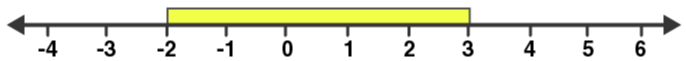B’ = {x ∈ R: 3 < x ≤ -4}

A ∩ B’ = {x ∈ R: 3 ≤ x < 5}

And this can be represented on number line as: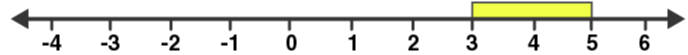15. Use real number line to find the range of values of x for which:

(i) x > 3 and 0 < x < 6

(ii) x < 0 and -3  x < 1

(iii) -1 < x  6 and -2  x  3

Solution:

(i) x > 3 and 0 < x < 6

Both the given inequations are true in the range where their graphs on the real number lines overlap.

And, the graphs of these inequations can be drawn as below:

x > 3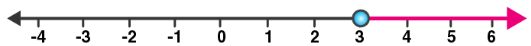0 < x < 6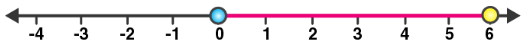Thus, from both the graphs, it is clear that their common range is

3 < x < 6

(ii) x < 0 and -3 ≤ x < 1

Both the given inequations are true in the range where their graphs on the real number lines overlap.

And, the graphs of these inequations can be drawn as below:

x < 0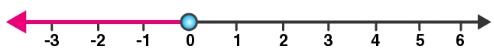-3 ≤ x < 1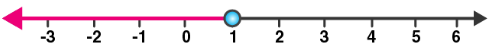Thus, from both the graphs, it is clear that their common range is

-3 ≤ x < 0

(iii) -1 < x ≤ 6 and -2 ≤ x ≤ 3

Both the given inequations are true in the range where their graphs on the real number lines overlap.

And, the graphs of these inequations can be drawn as below:

-1 < x ≤ 6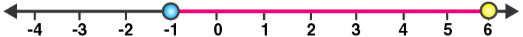-2 < x ≤ 3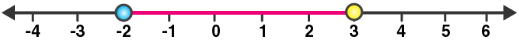Thus, from both the graphs, it is clear that their common range is

-1 < x ≤ 3

16. Illustrate the set {x: -3  x < 0 or x > 2, x  R} on the real number line.

Solution:

We have to understand that:

Graph of solution set of -3 ≤ x < 0 or x > 2 = Graph of points which belong to -3 ≤ x < 0 or x > 2 or both

Therefore, the required graph is as below: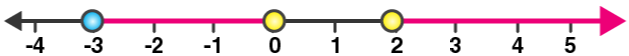17. Given A = {x: -1 < x ≤ 5, x ∈ R} and B = {x: -4 ≤ x < 3, x ∈ R}

Represent on different number lines:

(i) A ∩ B

(ii) A’ ∩ B

(iii) A – B

Solution:

(i) A ∩ B = {x: -1 < x < 3, x ∈ R}

And, it can be represented on a number line as: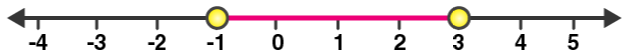(ii) Numbers which belong to B but do not belong to A = B – A

A’ ∩ B = {x: -4 ≤ x ≤ -1, x ∈ R}

And, it can be represented on a number line as: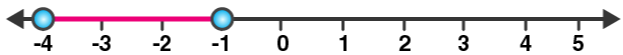(iii) A – B = {x: 3 ≤ x ≤ 5, x ∈ R}

And, it can be represented on a number line as: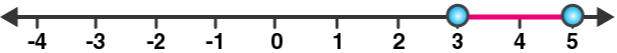18. P is the solution set of 7x – 2 > 4x + 1 and Q is the solution set of 9x – 45 ≥ 5(x – 5); where x  R. Represent:

(i) P  Q

(ii) P – Q

(iii) P  Q’ on different number lines.

Solution:

P = {x: 7x – 2 > 4x + 1, x ∈ R}

7x – 2 > 4x + 1

7x – 4x > 1 + 2

3x > 3

x > 1

And,

Q = {x: 9x – 45 ≥ 5(x -5), x ∈ R}

9x – 45 ≥ 5x – 25

9x – 5x ≥ 45 – 25

4x ≥ 20

x ≥ 5

(i) P ∩ Q = {x: x ≥ 5, x ∈ R }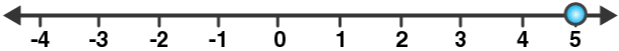(ii) P – Q = {x: 1 < x < 5, x ∈ R}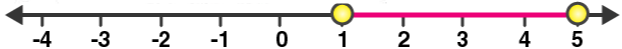(iii) P ∩ Q’ = {x: 1 < x < 5, x ∈ R }